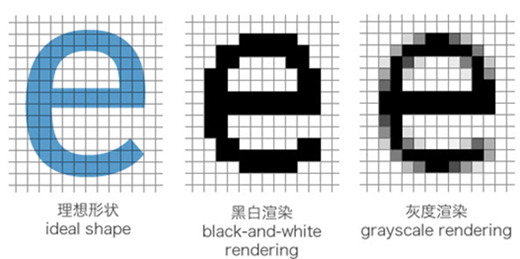Pandas+SQLAlchemy与数据库交互使用sqlalchemy连接数据库

Engine 翻译过来就是引擎的意思，汽车通过引擎来驱动，而 SQLAlchemy 是通过 Engine 来驱动，Engine 维护了一个连接池（Pool）对象和方言（Dialect）。方言简单而言就是你连的到底是 MySQL 还是 Oracle 或者 PostgreSQL 还是其它数据库。

from sqlalchemy import create_engine

engine = create_engine('sqlite:///test.db')
# engine = create_engine("mysql+pymysql://root:root@localhost/hackathon")

import urllib.parse
urllib.parse.quote_plus("kx%jj5/g")

将 DataFrame保存到数据库中

DataFrame自带to_sql方法，使用方法如下：

DataFrame.to_sql(self, name: str, con, schema=None, if_exists: str = 'fail', index: bool = True, index_label=None, chunksize=None, dtype=None, method=None)

• name：数据库表名称
• con：数据库连接
• schema：数据库模的schema，大部分连接的时候不需要
• if_exists：可选：{‘fail’, ‘replace’, ‘append’}
• index：是否将DataFrame的index列写入
• index_label：index列的名称
• chunksize：设置批量写入的条目数量
• dtype：设置字段类型
• method：插入方法，可不做设置

df.to_sql(con=engine, name='pair_result', if_exists='append')

传统方式：通过游标方式取数据库数据

with engine.connect() as con:
rs = con.execute('SELECT * FROM testdb.abc')
for row in rs:
print(row)

with engine.connect() as con:
rs = con.execute('SELECT * FROM testdb.abc ')
df = pd.DataFrame(rs.fetchall())
df.columns = rs.keys()

from sqlalchemy import create_engine
import pandas as pd

engine = create_engine('sqlite:///test.db')

# 查询表数据

# 使用SQL查询数据
query = '''SELECT *
FROM sub_place
WHERE father_area_name='中国'
'''